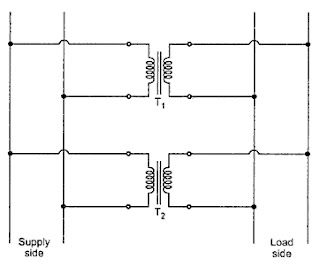### Parallel Operation of Transformers

Parallel Operation of Transformers
The transformers are connected in parallel when the load on them is more than the rating of the individual transformers. Several smaller units are operated in parallel which share a common load. Thus it is avoided that the total load is supplied by single unit due to use of parallel operation. The parallel operation is advantageous in the sense that the spare parts can be used interchangeably and their storage is easy.
From the Fig. 1. it can be seen that the primary windings are connected to the supply bus bars while the secondary windings are connected to load bus bars.Fig. 1  Parallel operation

Thus two or more transformers are connected in parallel to carry common load. If a given transformer is insufficient in capacity to deliver a particular load it may either be taken out of the circuit and replaced with a larger unit or an additional unit may be added to the circuit by connecting its primary side to the same source load circuit. The second unit is then said to be operating in parallel with the first unit. For satisfactory parallel operation of transformers there are certain conditions that must be satisfied.
The parallel operation may be obtained generally by connecting common primary and secondary leads and then connecting the bank thus formed to the primary and secondary circuits. This is shown in the Fig. 1.
The difference between the connection shown in the Fig.1 and the Fig. 2 is that, through both the transformers are energised from the same source of supply and are connected to the same load circuit, in first case the transformers are connected directly to the supply and load circuits whereas in second case the two units are first connected together and then connected to the respective supply and load circuits.Fig. 2    Alternative connection  of parallel transformer

The division of load between the two transformers connected in parallel by either of the two ways mentioned above will be different.
Key Point : Satisfactory parallel operation of the transformers implies that the transformers connected in parallel share common load approximately in proportion to their ratings. The most satisfactory condition is achieved when the load shared by the transformers is in exact proportion with their ratings.